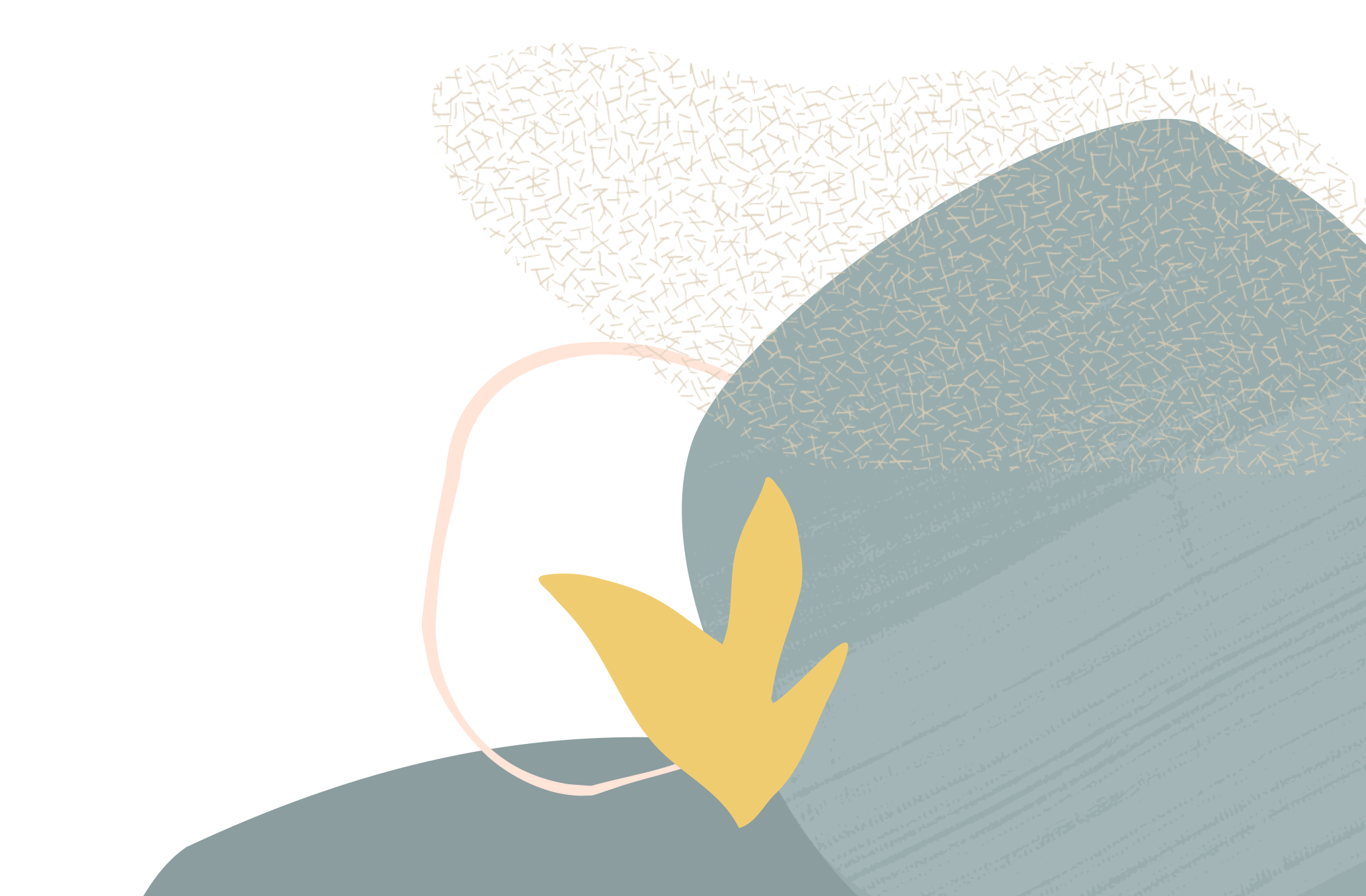35
Views
6

# Content

Hi,

I need to find greatest /least of two dates in OTBI using formula

how can we do this in OTBI?

Tagged:

• Hi - assuming that the two dates are the same field, then you would do MAX("FIELD").

• Hi - dates are in two different field

• Are the fields dates or timestamps? Either way, you should be able to use TIMESTAMPDIFF and some CASE statement. Pseudocode below:

`TIMESTAMPDIFF(SQL_TSI_DAY, TIMESTAMPADD(SQL_TSI_DAY, -7, CURRENT_DATE),TIMESTAMPADD(SQL_TSI_DAY, 0, CURRENT_DATE))`

...

CASE when (above formula >= 0) then date on right of method ELSE date on left of method END

Hope this helps.

• OK, try this then:

CASE
WHEN "FIELD1" > "FIELD2" THEN "FIELD1"
WHEN "FIELD2" > "FIELD1" THEN "FIELD2"
END

• Wait where's the ELSE? What if they're the same? :-P

• You do not always need the ELSE on there, in this statement it would just return a blank cell.

If you want it to return something for it being the same, you would do something like this:

CASE
WHEN "FIELD1" > "FIELD2" THEN "FIELD1"
WHEN "FIELD2" > "FIELD1" THEN "FIELD2"
ELSE 'SAME'
END

When I tested this, it errored because FIELD1 and FIELD2 are dates ... so I had to use code like this:

CASE
WHEN "FIELD1" > "FIELD2" THEN 'Field 1 name'
WHEN "FIELD2" > "FIELD1" THEN 'Field 2 name'
ELSE 'SAME'
END

I am not sure how to combine the text/date fields for it to work.